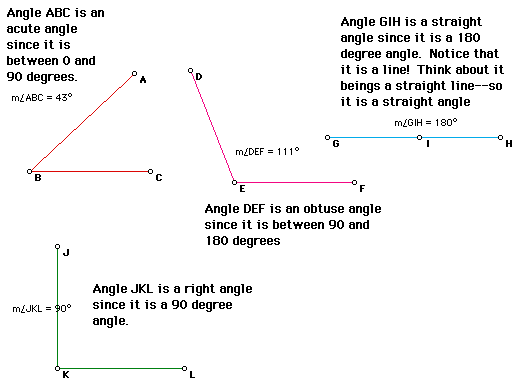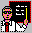 Types of Angles

# Types of Angles

In geometry, there are three types of angles:
1. acute angle-an angle between 0 and 90 degrees
2. right angle-an 90 degree angle
3. obtuse angle-an angle between 90 and 180 degrees
4. straight angle-a 180 degree angle

Here are a few examples of angles:Return to the Prerequisites Menu